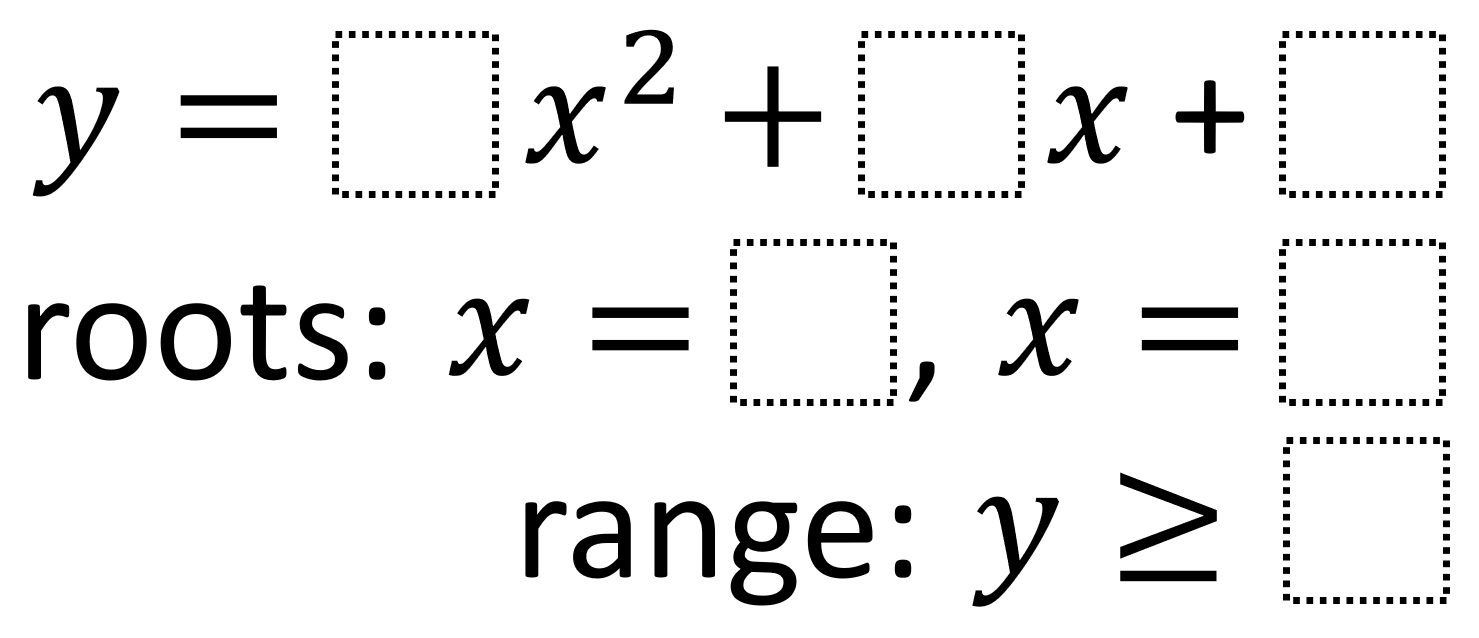# Trinomial Function Features 2

Directions: Using the integers -9 to 9, at most one time each, fill in the boxes to create a function with the corresponding range and roots that are as close together as possible.### Hint

How do each of the values you can choose affect the values of the roots and range?

The closest the two roots can be to each other that has been found so far is 2 from the equation:

y = 1x^2 + 6x + 8
roots: x = -4 and x = -2
range: y ≥ -1

Have you found an equations with roots that are even closer together? Share it in the comments.

Source: Robert Kaplinsky

## Period of Trig Function 3

Directions: Using the digits 0 to 9, at most one time, fill in the blanks …